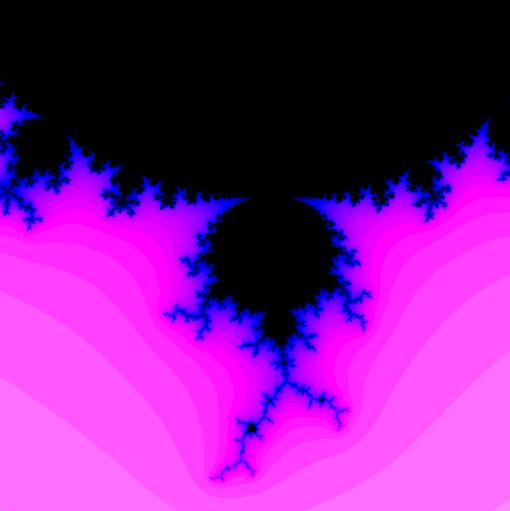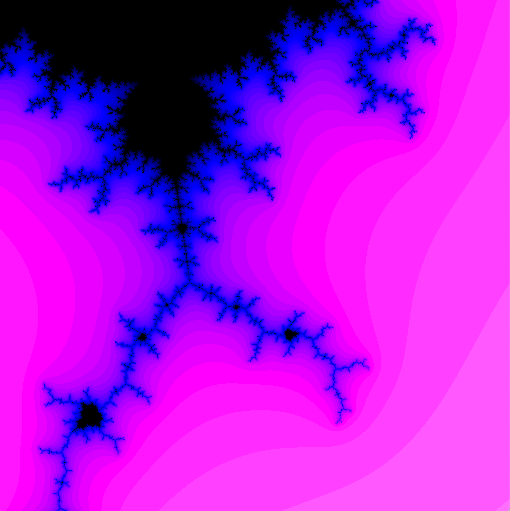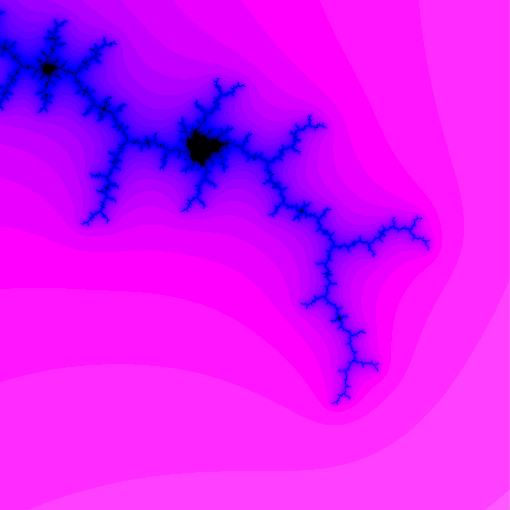Lab Week 05: Executors and Fractals

Announcements

1. I owe you feedback!
2. Homework 02 posted, due Friday
• fairly brief (3 questions)
3. Accountability groupsOutline

2. The Mandelbrot Set
3. Lab 03: Drawing the Mandelbrot Set

Previously

• Created Threads and ran them in parallel
• implmenet Runnable interface
• create and start instances
• join to wait until threads finish

Drawbacks

• best performance by balancing number of threads/processors available
• Need to explicitly partition into relatively few pieces
• partitioning may be unnatural
• partition may be unbalanced:
• don’t know in advance how long computations will take

When tasks are fairly homogenous (e.g., computing $\pi$, shortcuts) previous approach is good

A (Sometimes) Better Way

A nice Java feature: thread pools

• Create a (relatively small) pool of threads
• Assign tasks to the pool

• Fixed partition of problem may be unbalanced
• e.g., processing requests for web server

• Implement Executor interface
• void execute(Runnable command) method
• More control of task handling: ExecutorService interface:
• wait for tasks to complete
• shut down pool (don’t accept new tasks)

Built-in ExecutorService Implementations

From java.util.concurrent.Executors:

• make a pool with a fixed number of threads
• make a pool with a single thread
• make pool that creates new threads as needed (reuses old if available)

public class MyTask implements Runnable {
...
public void run () {
...
}
}

Create a pool, e.g., fixed thread pool

Shutting down the pool

pool.shutdown();

Wait for all pending processes to complete (like join() method)

try {

pool.awaitTermination(Long.MAX_VALUE, TimeUnit.NANOSECONDS);

} catch (InterruptedException e) {

// do nothing

}

Example

Shortcuts from Lab 02:

for (int i = 0; i < size; ++i) {
for (int j = 0; j < size; ++j) {

float min = Float.MAX_VALUE;

for (int k = 0; k < size; ++k) {
float x = matrix[i][k];
float y = matrix[k][j];
float z = x + y;

if (z < min) {
min = z;
}
}

shortcuts[i][j] = min;
}
}

For fixed row i, col j:

float min = Float.MAX_VALUE;

for (int k = 0; k < size; ++k) {
float x = matrix[i][k];
float y = matrix[k][j];
float z = x + y;

if (z < min) {
min = z;
}
}
shortcuts[i][j] = min;

Two Approaches

Approach 1:

• need size * size threads

Approach 2:

• Make a thread pool and let the pool decide
• choose pool size from availableProcessors()

Test Them

• Which method is faster? How much?
• How does pool performance compare to previous multithreaded version (without optimizing cache locality)?

The Mandelbrot Set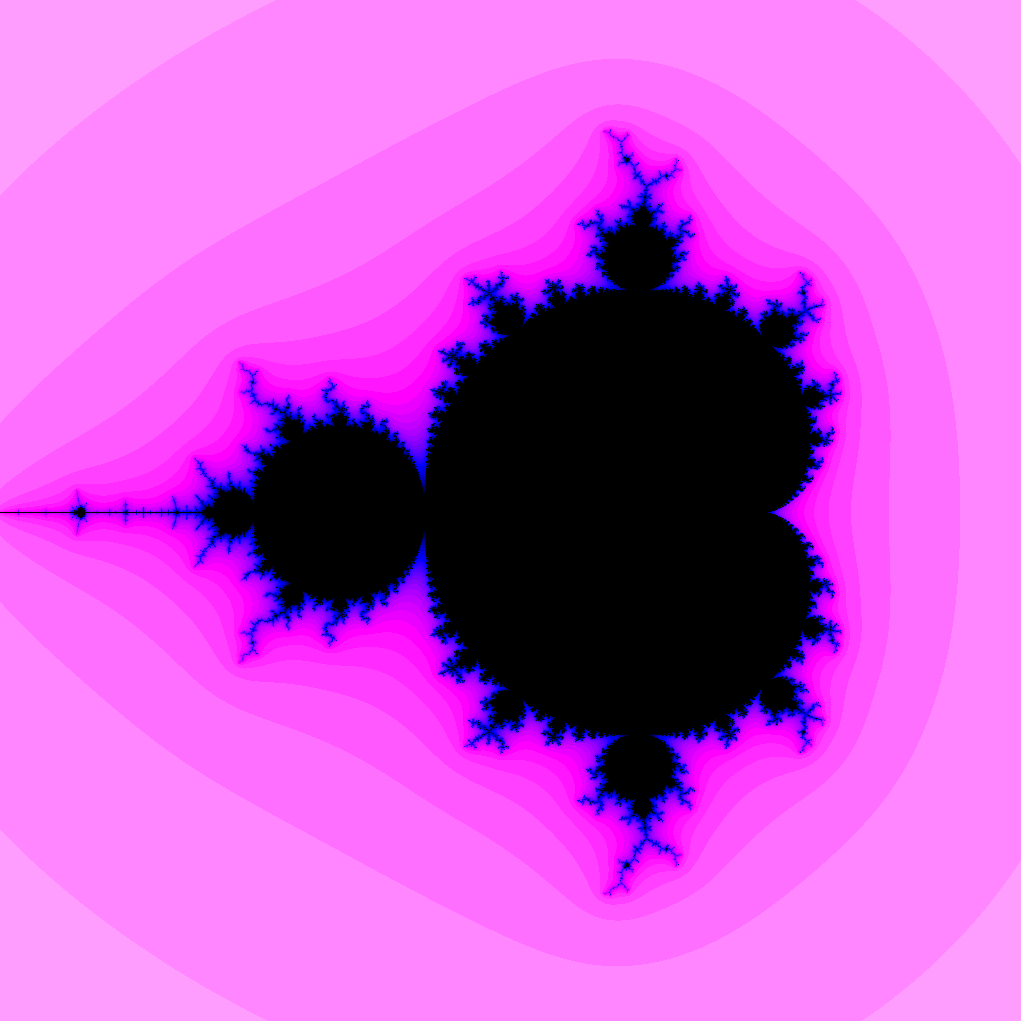Complex Numbers

Recall:

• the imaginary number $i$ satisfies $i^2 = -1$
• complex numbers are number of the form $a + b i$ where $a$ and $b$ are real
• complex arithmetic:
• $(a + b i) + (c + d i) = (a + c) + (b + d) i$
• $(a + b i) \cdot (c + d i) = (a c - b d) + (a d + b c) i$
• modulus (or length):
• $|a + b i| = \sqrt{a^2 + b^2}$

Complex Plane

Associate complex number $a + b i$ with point $(a, b)$ in plane

Iterated Operations

Fix a complex number $c$

• Define sequence $z_1, z_2, z_3, \ldots$ by
• $z_1 = c$
• for $n > 1$, $z_{n} = z_{n-1}^2 + c$
• What happens for different values of $c$?

Mandelbrot Set

The Mandelbrot set is the set of complex numbers $c$ such that the sequence $z_1, z_2, \ldots$ remains bounded (i.e., $|z_n|$ does not grow indefinitely)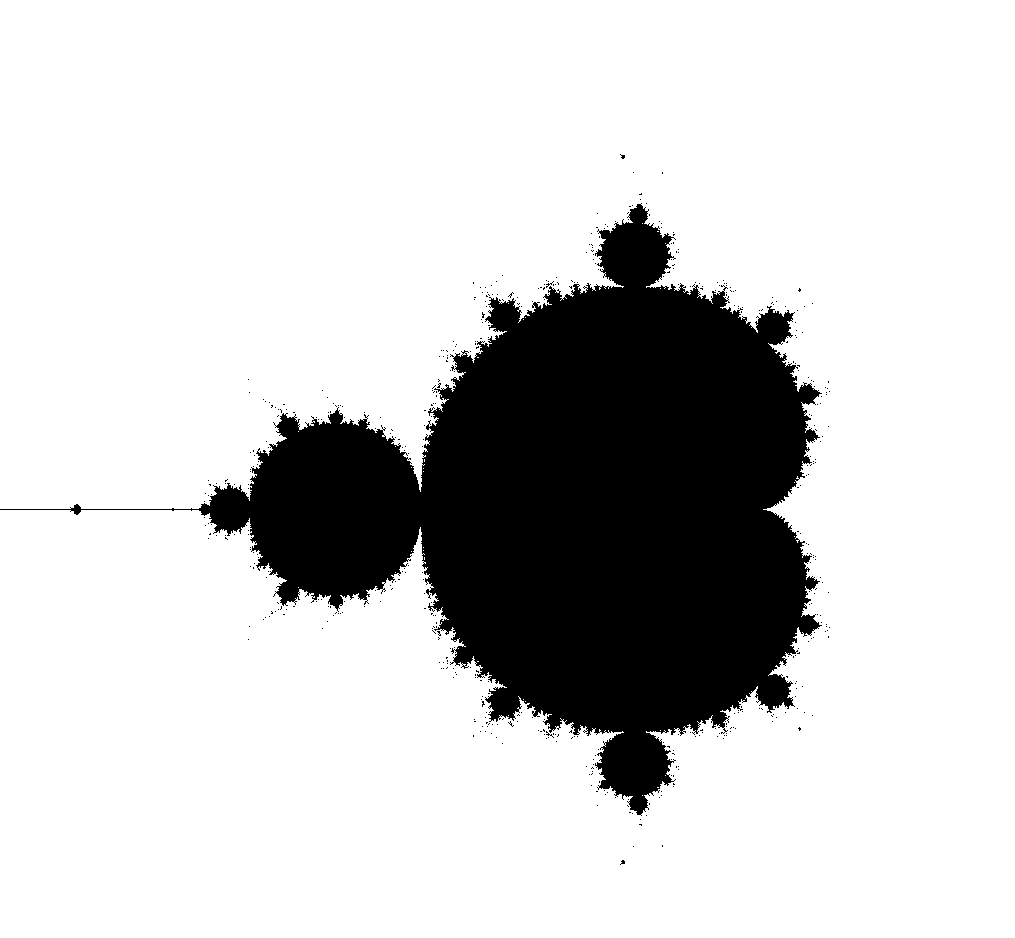Computing the Mandelbrot Set

Choose parameters:

• $N$ number of iterations
• $M$ maximum modulus

Given a complex number $c$:

• compute $z_1 = c, z_2 = z_1^2 + c,\ldots$ until
1. $|z_n| \geq M$
• stop because sequence appears unbounded
2. $N$th iteration
• stop because sequence appears bounded
• if $N$th iteration reached $c$ is likely in Mandelbrot set

Drawing the Mandelbrot Set

• Choose a region consisting of $a + b i$ with
• $x_{min} \leq a \leq x_{max}$
• $y_{min} \leq b \leq y_{max}$
• Make a grid in the region
• For each point in grid, determine if in Mandelbrot set
• Color accordingly

Counting Iterations

Given a complex number $c$:

• compute $z_1 = c, z_2 = z_1^2 + c,\ldots$ until
1. $|z_n| \geq M$
• stop because sequence appears unbounded
2. $N$th iteration
• stop because sequence appears bounded
• if $N$th iteration reached $c$ is likely in Mandelbrot set

Color by Escape Time

1. Color black in case 2 (point is in Mandelbrot set)
2. Change color based on $n$ in case 1:
• smaller $n$ are “farther” from Mandelbrot set
• larger $n$ are “closer”Partitions?